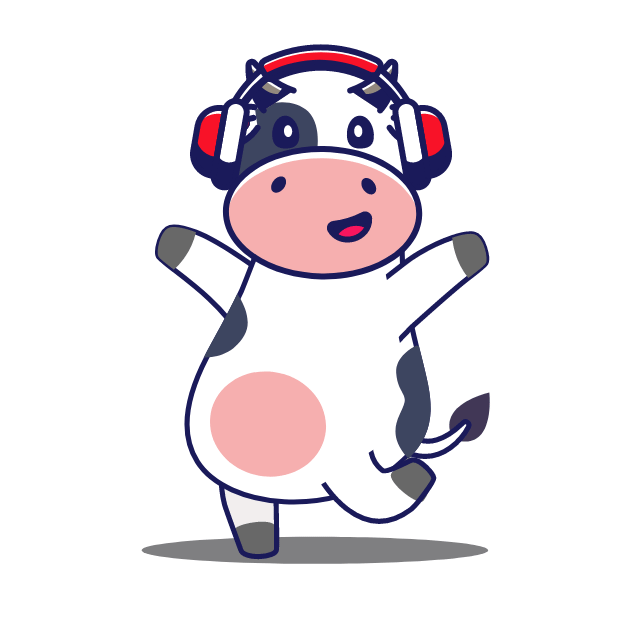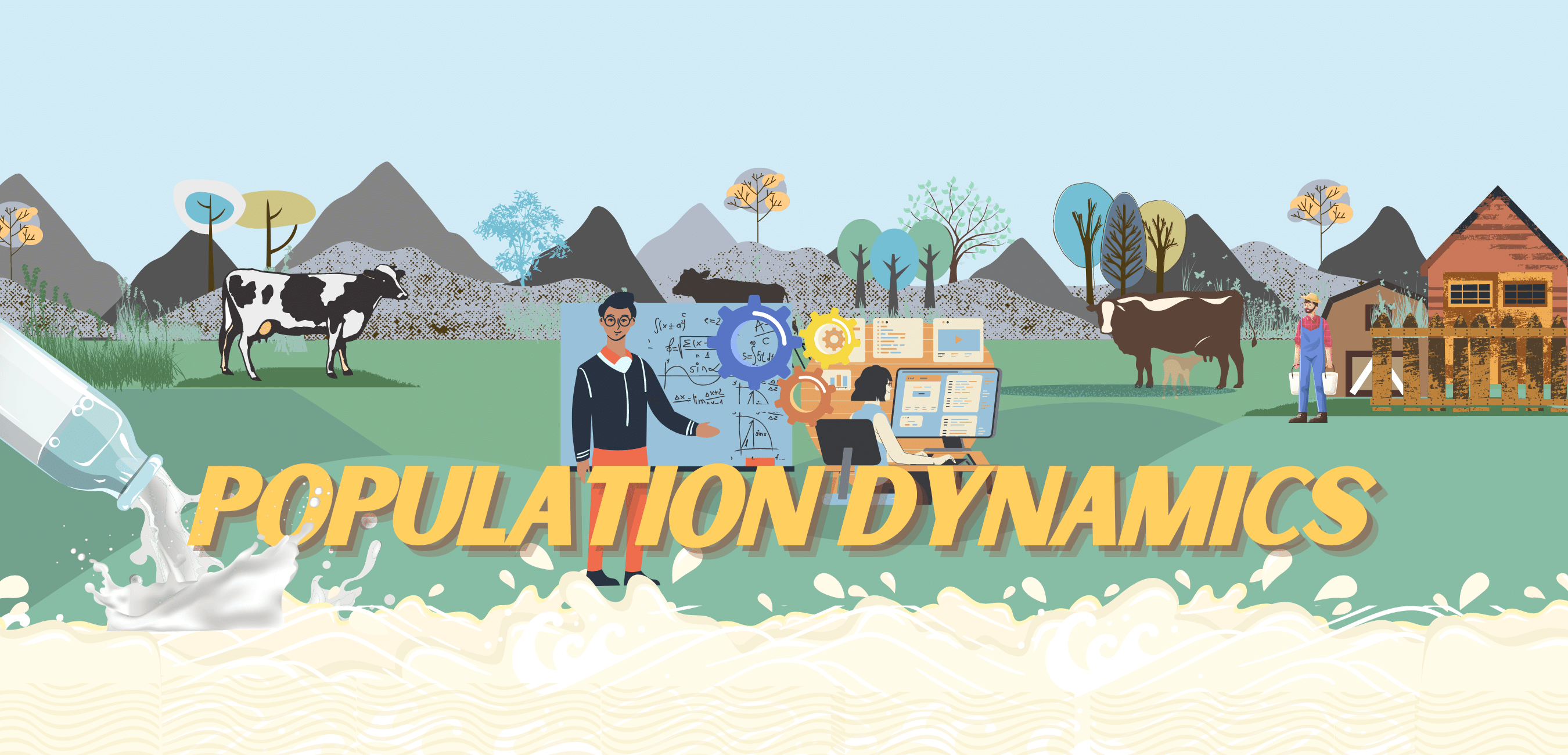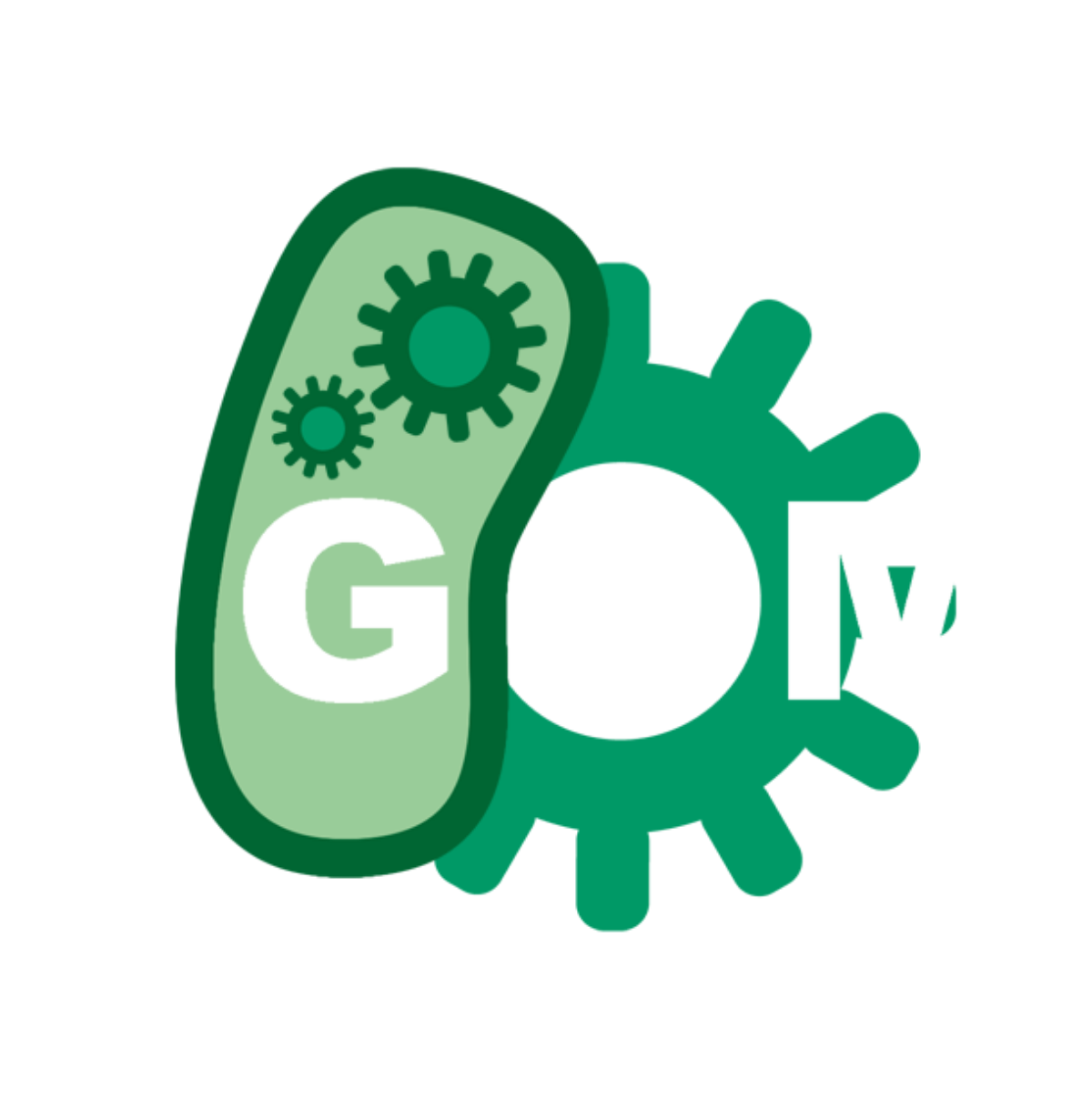# Team:IISER Kolkata/PopulationDynamicsLoading . . .Epidemiological Model for spread of bovine mastitis

Model Development

Bovine mastitis is a disease that spreads from cow to cow. Hence the understanding of its disease dynamics is based on the infectious disease modelling techniques that give us a more profound insight into the real world applications of the project. Moving forward with this, it is imperative to note that although all models involving spread of infectious diseases are simplistic approximations of real-world scenarios. However, they do provide us with crucial insights when large populations are involved and the law of large numbers come to play. Simplistic models allow us to explain analytically the influence of parameters on the occurrence of an epidemic, its size and duration. This is associated with the cost of sacrificing finer details that lead to real-world scenarios. At the other end of the spectrum, models can be developed that contain extensive detail about interactions at the individual level. However, due to the complexity of such models, interpretation is exceedingly challenging.

We have developed a stochastic SIR model using the network based Gillespie Algorithm. The network of choice defines the connection between agents through which the disease spreads.

The basic SIR model is as follows:

$$\dot{S(t)} = - \frac{\beta I(t) S(t)}{N}$$ $$\dot{I(t)} = \frac{\beta I(t) S(t)}{N} - \gamma I(t)$$ $$\dot{R(t)} = \gamma I(t)$$ $$N=S(0)+I(0)+R(0)$$

In the stochastic version of the SIR model, the continuous variables are discretised, and the process "rates" are replaced by process "probabilities". The transitions are given by-

$$(S,I,R) \xrightarrow{\frac{\beta I S}{N}} (S-1,I+1,R)$$ $$(S,I,R) \xrightarrow{\gamma I} (S,I-1,R+1)$$

Although conceptually, the stochastic SIR model is more difficult to implement than its deterministic counterpart, but that is not the case when it comes to computational simulations of the models. This is accounted by the fact that computers handle discrete variables with more ease than continuous ones. We simulated the stochasticity using a random number generator.

In the brute-force method to simulate the stochastic SIR model, we have to set exceedingly small time-steps in the simulation so that, at max, one process happens at a given step. This avoids technicalities arising from two or more processes happening simultaneously. However, this method is inefficient as nothing happens during the majority of the time steps. In the case of the Gillespie algorithm, the occurrence of the next event is calculated. Suppose the current time is t. The time $$t + \tau$$ at which something happens next is an exponentially distributed random number scaled by the sum of all process rates,$$\sum_{i} {a_i}$$ . Then, the Gillespie algorithm determines what happens next by drawing a process randomly from all possible processes with weighted probabilities.

At the early stage of an outbreak, classical models treat the immediate neighbourhood of an individual as fully susceptible. This tends to overestimate the spread of the outbreak. Furthermore, the neighbourhood of individuals infected later will tend to be less susceptible than for the region that the disease has already spread to. Therefore, the equations defining classical models may be inaccurate in later stages of the disease epidemiology. Keeping these aspects in mind, we have introduced a network-based modelling approach to overcome the aforementioned shortcomings of classical models.

Assumptions

In our model, we have used the Watts-Strogatz random graph model to mimic the real world scenario within a herd of cattle. It is a graph with small-world properties, short average path lengths and high clustering coefficients. We have taken 100 nodes and a 'k' value as 4 for our simulation. We have also associated it with a weighted graph where weights were generated randomly between 0 to 1.

In our stochastic network SIR model, we have taken the $$\beta$$ value (transmission factor) from a random distribution with values ranging from 0.64 to 0.72. We know that under treatment with the best available therapeutic, a diseased cow recovers within a time span of 5 to 7 days on average. In our proposed GMO based treatment the cow should recover within 3 to 5 days. Similarly, the $$\gamma$$ value used for both the models is also randomised between the range. We have started our simulation with an initial number of cases set at six individuals. We have not considered any birth or death in our model as it is a relatively very small time scale with respect to change in population.

Result and Analysis

In order to understand the practicality of our solution we tried to see the community structure in the graph and used greedy modularity community detection algorithm. Eight communities were identified in the graph which consisted of 100 nodes. This is in line with a practical scenario in which the typical size of a herd of cows ranges from 10-15 individuals.

From comparing both scenarios with the currently best possible antibiotic treatment and our proposed GMO based treatment we can infer that as our method treats the disease faster, it will be more effective. From the animations, we can see the outbreak size is smaller and lasts for a shorter amount of period for our proposed treatment.

Our detection kit actually enables early detection which will also decrease the size of the epidemic, but this component is not included in our simple modelling technique. We have used Network and EON python modules to implement our model.

Mathematical Model for Testing

Model Development

Suppose there are a total of 'S' samples to be tested for sub-clinical bovine mastitis. We will try to optimise the total number of tests needed to be performed to exactly ascertain which samples are infected with the disease. In the first round samples are tested by grouping them together. The results of the previous test samples are ascertained and the sample groups that have tested positive are isolated. These positive samples are tested individually. We will make a group of 'n' samples.

Let's assume p is the probability of the disease (testing positive) in all samples,

so (1-p) probability of the sample being tested negative.

So $$(1-p)^{n}$$ is the probability of all n samples in that group to be tested negative

Then $$1-(1-p)^{n}$$ is the probability of at least one of the samples being positive.

There are total $$\frac{S}{n}$$ groups and each group has n samples, so for the 2nd round $$S(1-(1-p)^{n})$$ test needed to be performed.

For the 1st round $$\frac{S}{n}$$ tests are needed to be performed. So total number of test are $$\frac{S}{n} + S(1-(1-p)^{n})$$.

We will take 'p' and 'S' as a fixed input and optimize the total number of tests to be performed as a function of 'n'.

Result and Analysis

Performing the 1-D minimization with several values of 'S' and 'p', we found that when the p-value is more than 0.3, the grouping strategy does not yield good results and testing all individuals is the best policy. Also for a herd of 100 to 500 cows, we found under p=0.3, grouping with 3 samples provide the best results. For a very large scale testing, it will be better rather than random groupings, if nearby and contact cows of a disease infected cows are grouped along with our strategy to lower the number of tests needed to finally conclude disease is positive or negative in cases of all the cows.

### References

1. I. Z. Kiss, J. C. Miller, and P. Simon,(Book) Mathematics of epidemics on networks: from exact to approximate models. Springer, 2017.
2. S. Schafroth and R. R. Regoes, “Stochastic simulation of a simple epidemic.”
3. P. Down, M. Green, and C. Hudson, “Rate of transmission: A major determinant of the cost of clinical mastitis,”Journal of Dairy Science, vol. 96, no. 10, pp. 6301–6314, 2013